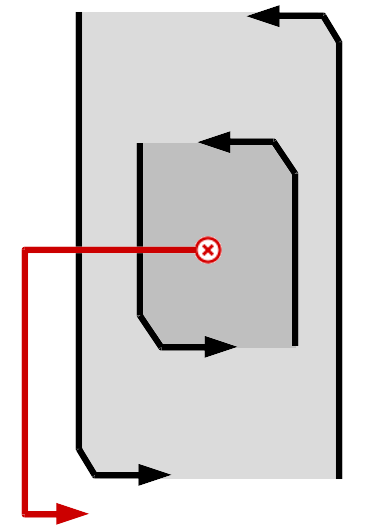# Programming-Idioms#### History of Idiom 43 > diff from v52 to v53

Edit summary for version 53 :
[C] avoiding goto statement

#### Version 52

2020-03-03, 14:23:27

#### Version 53

2020-03-03, 14:24:32

# Idiom #43 Break outer loop

Look for a negative value v in 2D integer matrix m. Print it and stop searching.# Idiom #43 Break outer loop

Look for a negative value v in 2D integer matrix m. Print it and stop searching.##### Imports
`#include <stdio.h>`
##### Imports
`#include <stdio.h>`
##### Code
```int i,j;
for(i=0;i<sizeof(m)/sizeof(*m);i++)
{
for(j=0;j<sizeof(*m)/sizeof(**m);j++)
{
if(m[i][j]<0)
{
printf("%d\n",m[i][j]);
i=pow(i,4);
break;
}
}
}
end:```
##### Code
```int i,j;
for(i=0;i<sizeof(m)/sizeof(*m);i++)
{
for(j=0;j<sizeof(*m)/sizeof(**m);j++)
{
if(m[i][j]<0)
{
printf("%d\n",m[i][j]);
i=sizeof(m)/sizeof(*m);
break;
}
}
}
end:```